Courses
Courses for Kids
Free study material
Free LIVE classes
More

# NCERT Solutions for Class 9 Maths Chapter 8 - ExerciseLIVE
Join Vedantu’s FREE Mastercalss

## Class 9 Maths Chapter 8 Quadrilaterals Exercise 8.1

For the students who are enthusiastic about learning Mathematics to gain in-depth knowledge, the revision notes of NCERT Solutions for Class 9 Maths Exercise 8.1 will become a good companion. Not only explaining chapter by chapter for every class, but the notes have also provided a detailed explanation for every sum in the exercise. Vedantu offers NCERT Solutions Class 9 Maths to students so that they can develop a strong foundation for this subject.These CBSE Solutions are very helpful for learners who aim to understand every problem of the chapter. The download option is also available for these notes on the official website of Vedantu. Students can also avail of NCERT Solutions for Class 9 Science from our website.

Last updated date: 29th Sep 2023
Total views: 514.2k
Views today: 15.14k
Watch videos on
NCERT Solutions for Class 9 Maths Chapter 8 - ExerciseQuadrilaterals Full Chapter in One Shot | CBSE Class 9 Maths Chapter8 | Term2 | Umang 2021 - Vedantu
Vedantu 9&10
SubscribeShare
5.7K likes
160.8K Views
1 year ago
Play QuizQuadrilaterals L-2( Mid Point Theorem ) | CBSE Class 9 Maths Chapter 8 | Term 2 Preparation 2022
Vedantu 9&10
3.9K likes
100.3K Views
1 year agoQuadrilaterals L-1( Properties of Quadrilaterals ) CBSE Class 9 Maths Chapter 8 | Term 2 Preparation
Vedantu 9&10
7.9K likes
175.5K Views
1 year ago
Play QuizEasy Way To Learn QUADRILATERALS | Vedantu Class 9 | Maths Chapter 8 | NCERT Solutions
Vedantu 9&10
5.5K likes
202.9K Views
4 years ago

## Access NCERT solution for Class-9 Maths Chapter 8 – Quadrilateral

### EXERCISE NO: 8.1

1. The angles of quadrilateral are in the ratio $3:5:9:13$. Find all the angles of the quadrilateral.

Ans: Given that the ratio of the angles of a quadrilateral is $3:5:9:13$.

Let us assume the common ratio between the angle of quadrilateral as $x$.

Now the angles of the quadrilateral are $3x$, $5x$, $9x$ and $13x$ respectively.

We know that the sum of the angle of the quadrilateral is $360{}^\circ$. Hence, we can write

$3x+5x+9x+13x=360{}^\circ$

$\Rightarrow 30x=360{}^\circ$

$\Rightarrow x=\dfrac{360{}^\circ }{30}$

$\Rightarrow x=12{}^\circ$

Now the value of the angles by using the common ratio value are given by

$3x=3\left( 12{}^\circ \right)=36{}^\circ$

$5x=5\left( 12{}^\circ \right)=60{}^\circ$

$9x=9\left( 12{}^\circ \right)=108{}^\circ$

$13x=13\left( 12{}^\circ \right)=156{}^\circ$

Hence the angles of the quadrilateral are $36{}^\circ$, $60{}^\circ$, $108{}^\circ$ and $156{}^\circ$.

2. If the diagonals of a parallelogram are equal, then show that it is a rectangle.

Ans: Given that the diagonals of a parallelogram are equal. Let us assume a parallelogram $ABCD$ whose diagonals are equal as shown in the figure below.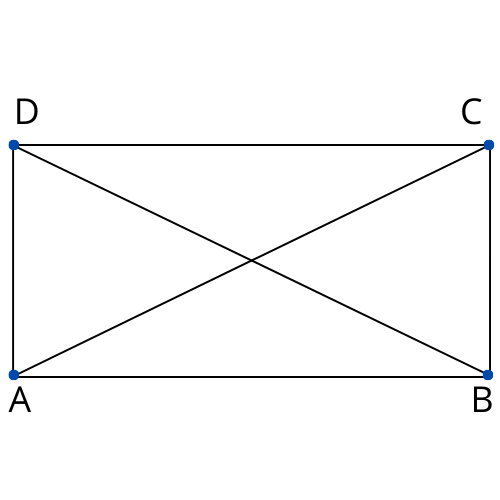Here $ABCD$ is a parallelogram, so we can write that $AB=DC$ since opposite sides of a parallelogram are equal.

Consider the triangles $ABC$ and $DCB$. In these two triangles we have $AB=DC$, $BC=BC$ and as per given data $AC=DB$. So, we can write that $\Delta ABC\cong \Delta DCB$ by SSS Congruence rule.

$\Rightarrow \angle ABC=\angle DCB$

We know that the sum of the angles on the same side of the transversal is $180{}^\circ$.

$\therefore \angle ABC+\angle DCB=180{}^\circ$

Substitute the value $\angle ABC=\angle DCB$ in the above equation.

$\Rightarrow \angle ABC+\angle ABC=180{}^\circ$

$\Rightarrow 2\angle ABC=180{}^\circ$

$\Rightarrow \angle ABC=\dfrac{180{}^\circ }{2}$

$\Rightarrow \angle ABC=90{}^\circ$

Here we have the angle $ABC$ and $DCB$ as $90{}^\circ$. When the angles of the parallelogram are equal to $90{}^\circ$, then it is called as Rectangle.

Hence, if the diagonals of the parallelogram are equal then it is called as Rectangle.

3. Show that if the diagonals of a quadrilateral bisect each other at right angles, then it is a rhombus.

Ans: Given that the diagonals of a quadrilateral bisect each other at right angles.

Let $ABCD$ be the quadrilateral, whose diagonals are bisecting each other at right angle. The diagram of the quadrilateral $ABCD$ is given below.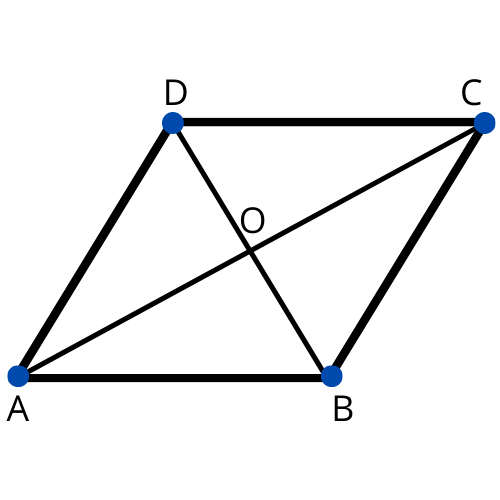In the above quadrilateral the diagonals bisect each other. So, we can write that $OA=OC$, $OB=OD$ and $\angle AOB=\angle BOC=\angle COD=\angle AOD=90{}^\circ$.

Consider $\Delta AOD$ and $\Delta COD$. In both the triangles we can write

$OA=OC$,

$\angle AOD=\angle COD$

$OD=OD$.

By using SAS congruence rule, we can write that $\Delta AOD\cong \Delta COD$. So,

$AD=CD$  by CPCT

Similarly, we can prove that

$AD=AB$ and $CD=BC$.

Finally, we can write that $AB=BC=CD=AD$.

Here we have opposite sides of the quadrilateral $ABCD$ are equal, so it will be a parallelogram. Again in $ABCD$ we have all sides equal, so it can be a Rhombus also.

4. Show that the diagonals of a square are equal and bisect other at right angles.

Ans: Consider a square $ABCD$ whose diagonals are $AC$ and $BD$. The intersecting point of the diagonals will be $O$ as shown in below figure.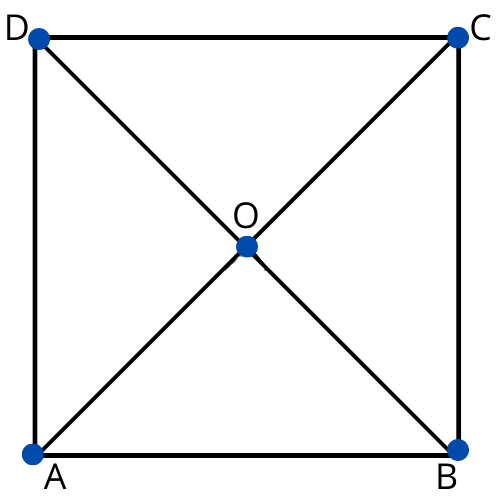According to the given statement we need to prove that $AC=BD$, $OA=OC$, $OB=OD$ and $\angle AOB=90{}^\circ$.

Consider $\Delta ABC$ and $\Delta DCB$.

In both the triangles we can write

$AB=DC$ since the sides of a square are equal to each other, $\angle ABC=\angle DCB=90{}^\circ$ and

$BC=CB$.

So, by using SAS congruency law we can say that $\Delta ABC\cong \Delta DCB$.

$\therefore AC=DB$ by CPCT.

Hence, the diagonals of the square are equal in length.

Now consider $\Delta AOB$ and $\Delta COD$.

In both the triangles we can write

$\angle AOB=\angle COD$ since vertically opposite angles are equal,

$\angle ABO=\angle CDO$ since alternate interior angles are also equal and

$AB=CD$ since the sides of a square are always equal.

So, by using AAS congruence rule we can say that $\Delta AOB\cong \Delta COD$.

$\therefore AO=CO$ and $OB=OD$ by CPCT.

Now consider $\Delta AOB$ and $\Delta COB$,

As we have proved earlier $AO=CO$,

$AB=CB$ since sides of a square are equal,

$BO=BO$.

By using SSS congruency rule we can write that $\Delta AOB\cong \Delta COB$.

$\therefore \angle AOB=\angle COB$ by CPCT.

But the angles $AOB$ and $COB$ are linear pair.

$\therefore \angle AOB+\angle COB=180{}^\circ$

$2\angle AOB=180{}^\circ$

$\angle AOB=90{}^\circ$

Hence, the diagonals of a square bisect each other at right angles.

5. Show that if the diagonals of a quadrilateral are equal and bisect each other at right angles, then it is a square.

Ans: Consider an equilateral $ABCD$ whose diagonals are $AC$ and $BD$. The intersecting point of the diagonals will be $O$ as shown in below figure.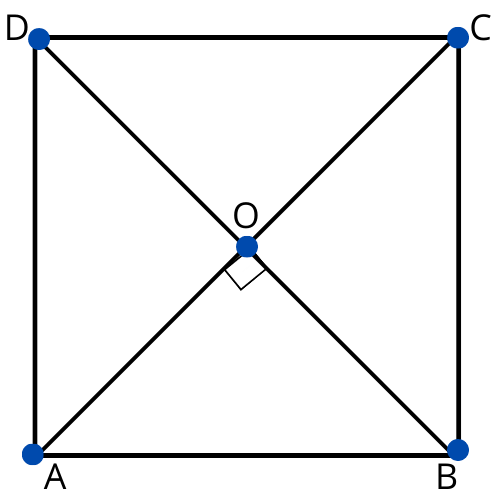According to the given statement we can write that $AC=BD$, $OA=OC$, $OB=OD$ and $\angle AOB=\angle BOC=\angle COD=\angle AOD=90{}^\circ$.

Consider $\Delta AOB$ and $\Delta COD$. In these two triangles we can write that

$AO=CO$ since diagonals bisect each other,

$OB=OD$ since diagonals bisect each other,

$\angle AOB=\angle COD$ since vertically opposite angles.

By using SAS congruence rule, we can say that $\Delta AOB\cong \Delta COD$.

$\therefore AB=CD$ and $\angle OAB=\angle OCD$ by CPCT.

However, these are alternate interior angles for line $AB$ and $CD$ However alternative interior angles are equal to each other only when the two lines are parallel.

$AB\parallel CD$

From all the above proofs we can say that $ABCD$ is a parallelogram.

Consider $\Delta AOD$ and $\Delta COD$, in these two triangles we can write that

$AO=CO$ since diagonals bisect each other,

$\angle AOD=\angle COD=90{}^\circ$,

$OD=OD$.

By using SAS congruence rule, we can say that $\Delta AOD\cong \Delta COD$.

$\therefore AD=DC$ by CPCT

But $ABCD$ is a parallelogram so we can write that $AB=BC=CD=DA$.

That means all sides of the quadrilateral $ABCD$ are equal.

Consider $\Delta ADC$ and $\Delta BDC$, in these two triangles we can write that

$AD=BC$, $AC=BD$ and $DC=CD$.

By using SSS congruence rule, we can say that $\Delta ADC\cong \Delta BCD$.

$\therefore \angle ADC=\angle BCD$ by CPCT.

But we know that the angles $ADC$ and $BCD$ are co interior angles.

$\therefore \angle ADC+\angle BCD=180{}^\circ$

$\Rightarrow 2\angle ADC=180{}^\circ$

$\Rightarrow \angle ADC=90{}^\circ$

Here the interior angles of the quadrilateral are right angles.

So, for a $ABCD$ parallelogram the sides are equal to each other and all angles are right angles.

Hence $ABCD$ is a square.

6. Diagonal $AC$ of a parallelogram $ABCD$ bisects $\angle A$ as shown in figure.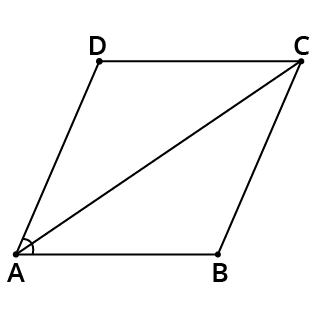• Show that it bisects $\angle C$.

Ans: Given that $ABCD$ is a parallelogram.

$\therefore \angle DAC=\angle BCA$ and $\angle BAC=\angle DCA$ since alternative interior angles

But in the problem, we have given that $AC$ bisect $\angle A$.

$\therefore \angle DAC=\angle BAC$

From all the above equations we can write that

$\angle DAC=\angle BCA=\angle BAC=\angle DCA$

$\angle DCA=\angle BAC$

Hence, $AC$ bisects $\angle C$.

• Show that $ABCD$ is a rhombus.

Ans: From the equation $\angle DAC=\angle BCA=\angle BAC=\angle DCA$, we can write that $\angle DAC=\angle DCA$.

$DA=DC$ since side opposite to equal angles are equal.

But we have $DA=BC$ and $AB=CD$ since opposite sides of the parallelogram are equal.

$\therefore AB=BC=CD=DA$

7. A rhombus $ABCD$ is given. Show that diagonal $AC$ bisects $\angle A$ as well as $\angle C$ and diagonal $BD$ bisects $\angle B$ as well as $\angle D$.

Ans: Let the diagram of the given rhombus will be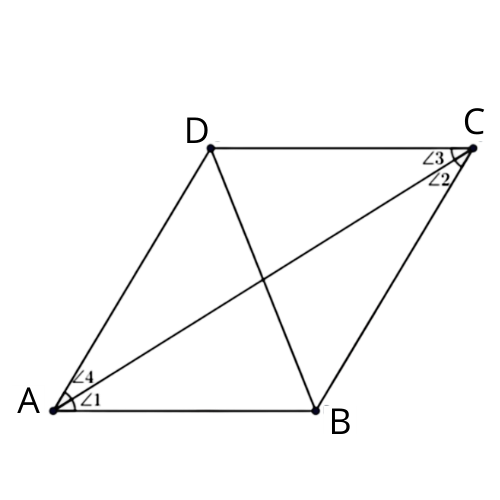Consider $\Delta ABC$, in this triangle we can write that

$BC=AB$ since sides of a rhombus are equal.

$\therefore \angle 1=\angle 2$ since angles opposite to equal sides of a triangle are equal.

But we have $\angle 1=\angle 3$ because they are alternate interior angles for parallel lines $AB$ and $CD$.

$\therefore \angle 2=\angle 3$

Hence the diagonal $AC$ bisects $\angle A$.

Also, $\angle 2=\angle 4$ since these two angles are also alternate interior angles for the parallel lines $BC$ and $DA$.

$\therefore \angle 1=\angle 4$

Hence the diagonal $AC$ bisects $\angle C$.

Similarly, we can prove the same for the diagonal $BD$.

8. $ABCD$ is a rectangle in which diagonal $AC$ bisects $\angle A$ as well as $\angle C$.

• $ABCD$ is a square.

Ans: Let the diagram of the given $ABCD$ rectangle is given by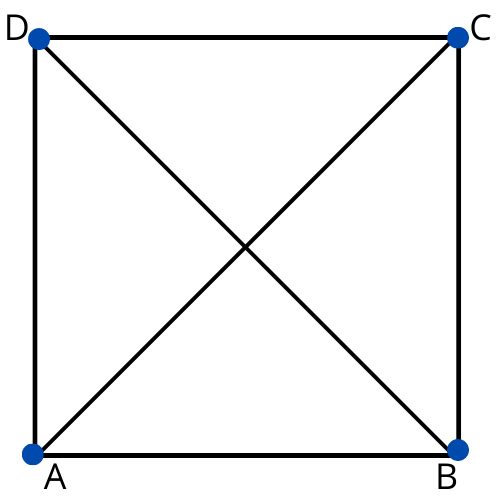We have given that $ABCD$ is a rectangle. So, we can write that

$\angle A=\angle C$

$\Rightarrow \dfrac{1}{2}\angle A=\dfrac{1}{2}\angle C$

But they have mentioned that $AC$ bisects $\angle A$ as well as $\angle C$.

$\Rightarrow \angle DAC=\dfrac{1}{2}\angle DCA$

$\therefore CD=DA$ since sides opposite to equal angles are also equal.

But $DA=BC$ and $AB=CD$ since opposite sides of a rectangle are equal.

$\therefore AB=BC=CD=DA$

Here $ABCD$ is given to be a rectangle but all the sides are equal.

Hence $ABCD$ is a square.

• Diagonal $BD$ bisects $\angle B$ as well as $\angle D$.

Ans: Now consider $\Delta BCD$, in this triangle we can write that

$BC=CD$ since sides of a square are equal,

$\angle CDB=\angle CBD$ since angles opposite to equal sides are equal.

But we have $\angle CDB=\angle ADB$ since alternate interior angles for parallel lines $AB$ and $CD$.

$\therefore \angle CBD=\angle ABD$

Hence $BD$ bisects $\angle B$.

Also, $\angle CBD=\angle ADB$ which are alternate interior angles for parallel lines $BC$ and $AD$.

$\therefore \angle CDB=\angle ADB$

Finally, $BD$ bisects $\angle D$ and $\angle B$.

9. In parallelogram $ABCD$, two points $P$ and $Q$ are taken on diagonal $BD$ such that $DP=BQ$ as shown in below figure.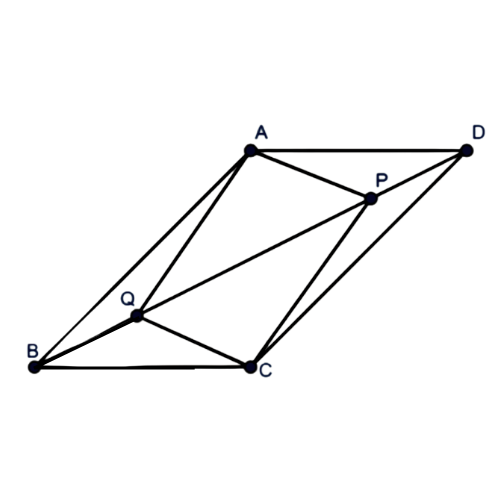• Show that $\Delta APD\cong \Delta CQB$

Ans: Consider $\Delta APD$ and $\Delta CQB$, in these two triangles we can write

$\angle ADP=\angle CBQ$ since alternate interior angles for the parallel lines $BC$ and $AD$,

$AD=CB$ since opposite sides of parallelogram are equal.

$DP=BQ$ which is in the given data.

By using SAS congruence rule, we can say that $\Delta APD\cong \Delta CQB$

• Show that $AP=CQ$

Ans: As we have $\Delta APD\cong \Delta CQB$. By using CPCT we can write that $AP=CQ$.

• Show that $\Delta AQB\cong \Delta CPD$

Ans: Consider $\Delta AQB$ and $\Delta CPD$, in these two triangles we can write

$\angle ABQ=\angle CDP$ since alternate interior angles for the parallel lines $AB$ and $CD$,

$AB=CD$ since opposite sides of parallelogram are equal.

$BQ=DP$ which is in the given data.

By using SAS congruence rule, we can say that $\Delta AQB\cong \Delta CPD$

• Show that $AQ=CP$

Ans: As we have $\Delta AQB\cong \Delta CPD$. By using CPCT we can write that $AQ=CP$.

• Show that $APCQ$ is a Parallelogram.

Ans: From the results obtained in (ii) and (iv), which are $AQ=CP$ and $AP=CQ$. That means the opposite sides of the quadrilateral $APCQ$ are equal, so $APCQ$ is a parallelogram.

10. $ABCD$ is a parallelogram and $AP$ and $CQ$ are perpendiculars from vertices $A$ and $C$ on diagonal $BD$ as shown in figure.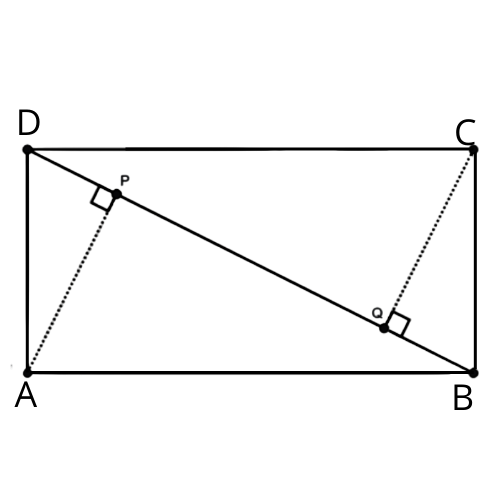• Show that  $\Delta APB\cong \Delta CQD$

Ans: Consider $\Delta APB$ and $\Delta CQD$, in these two triangles we can write that

$\angle APB=\angle CQD=90{}^\circ$,

$AB=CD$ since opposite sides of parallelogram,

$\angle ABP=\angle CDQ$ since alternate interior angles for parallel lines $AB$ and $CD$.

By using AAS congruency rule we can say that $\Delta APB\cong \Delta CQD$.

• Show that $AP=CQ$

Ans: From the statement $\Delta APB\cong \Delta CQD$, by using CPCT we can write that $AP=CQ$.

11. In $\Delta ABC$ and $\Delta DEF$, $AB=DE$, $AB\parallel DE$, $BC=EF$ and $BC\parallel EF$. Vertices $A$, $B$ and $C$ are joined to vertices $D$, $E$ and $F$ respectively as shown in figure.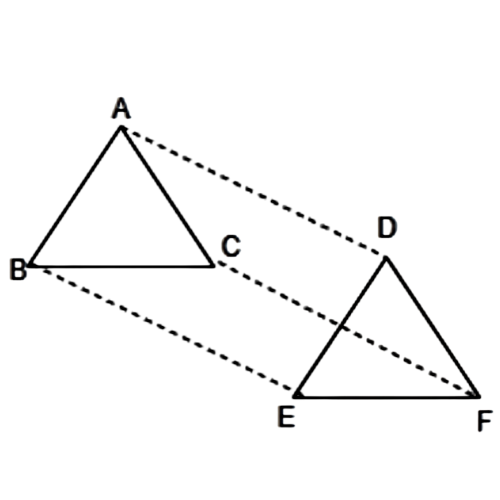• Show that quadrilateral $ABED$ is a parallelogram.

Ans: Given that $AB=DE$ and $AB\parallel DE$.

If two opposite sides of a quadrilateral are equal and parallel to each other, then it is called as parallelogram.

Hence quadrilateral $ABED$ is a parallelogram.

• Show that quadrilateral $BEFC$ is a parallelogram.

Ans: We have also given $BC=EF$ and $BC\parallel EF$.

Hence quadrilateral $BEFC$ is a parallelogram.

• Show that $AD\parallel CF$ and $AD=CF$

Ans: We have the parallelograms $ABED$ and $BEFC$. So, we can write that $AD=BE$, $AD\parallel BE$ and $BE=CF$, $BE\parallel CF$ since opposite sides of a parallelogram are equal and parallel.

$\therefore AD=CF$ and $AD\parallel CF$

• Show that quadrilateral $ACFD$ is a parallelogram

Ans: As we had observed that one pair of opposite sides ($AD$ and $CF$) of quadrilateral $ACFD$ are equal and parallel to each other, hence it is a parallelogram.

• Show that $AC=DF$

Ans: As $ACFD$ is a parallelogram, so the pair of opposite sides will be equal and parallel to each other.

$\therefore AC=DF$ and $AC\parallel DF$.

• Show that $\Delta ABC\cong \Delta DEF$

Ans: Consider $\Delta ABC$ and $\Delta DEF$, in these two triangles we have

$AB=DE$, $BC=EF$ and $AC=DF$.

By using SSS congruence rule we can say that $\Delta ABC\cong \Delta DEF$.

12. $ABCD$ is a trapezium on which $AB\parallel CD$ and $AD=BC$ as shown in figure. (Hint: Extend $AB$ and draw a line through $C$ parallel to $DA$ intersecting $AB$ at point $E$).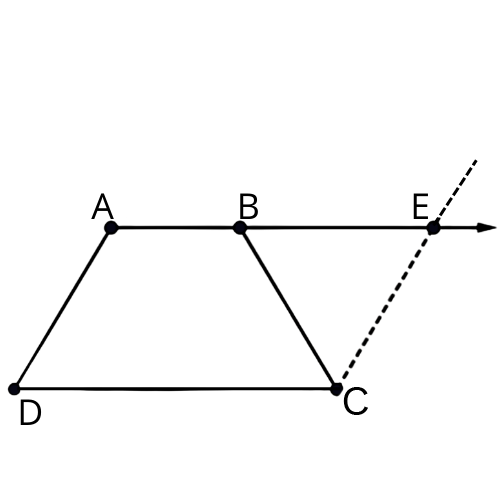• Show that $\angle A=\angle B$

Ans: We have $AD=CE$ opposite sides of parallelogram and $AD=BC$.

$\therefore BC=CE$ and $\angle CEB=\angle CBE$ since angle opposite to equal sides are also equal.

Consider the parallel lines $AD$ and $CE$. $AE$ is the transversal line for them.

So,

$\angle A+\angle CEB=180{}^\circ$ since angles on the same side of transversal.

$\Rightarrow \angle A+\angle CBE=180{}^\circ$

Also, $\angle B+\angle CBE=180{}^\circ$ as they are linear pair of angles. So, from these two equals we can write that

$\angle A=\angle B$

• Show that $\angle C=\angle D$

Ans: Given $AB\parallel CD$, so

$\angle A+\angle D=180{}^\circ$ , $\angle C+\angle B=180{}^\circ$since angles on the same side of the transversal.

$\therefore \angle A+\angle D=\angle C+\angle B$

But we have $\angle A=\angle B$, so we will have

$\angle C=\angle D$

• Show that $\Delta ABC\cong \Delta BAD$

Ans: Consider $\Delta ABC$ and $\Delta BAD$, in these two triangles we can write that

$AB=BA$, $BC=AD$ and $\angle B=\angle A$.

By using SAS congruence rule, we can say that $\Delta ABC\cong \Delta BAD$.

• Show that diagonal $AC=\text{ diagonal }BD$

Ans: We have $\Delta ABC\cong \Delta BAD$, so by using CPCT we can write that $AC=BD$

### CBSE Class 9 Maths Chapter 8 Exercise 8.1 Solutions free PDF

It is very easy to download the PDF format of NCERT Solutions for Class 9 Maths Exercise 8.1 from the official website of Vedantu for both parents and students. These can be downloaded for free and can be stored for future use. Students can revise these notes during the time of examinations without bothering about the internet connection.

### Important Concepts Covered in Exercise 8.1 of Class 9 Maths NCERT Solutions

Exercise 8.1 of Class 9 Maths NCERT Solutions is mainly focused on the below topics.

• Different types of quadrilaterals (a figure that is formed by joining four points in order is called a quadrilateral)

• Properties of the quadrilaterals (especially those of parallelograms such as the diagonal of a parallelogram and its sides)

Exercise 8.1 of Class 9 Maths NCERT Solutions consists of questions related to some special properties of quadrilaterals. These properties are listed below.

• A diagonal of a parallelogram divides it into two congruent triangles.

• In a parallelogram, opposite sides are equal.

• If each pair of opposite sides of a quadrilateral is equal, then it is a parallelogram.

• In a parallelogram, opposite angles are equal. If in a quadrilateral, each pair of opposite angles is equal, then it is a parallelogram.

• The diagonals of a parallelogram bisect each other. If the diagonals of a quadrilateral bisect each other, then it is a parallelogram.

It is advisable for the students to memorize all the definitions, theorems, and proof of theorems before solving the questions provided in Exercise 8.1. Practising these questions will be helpful for the students to have a better understanding of these key concepts.

### Exercise 8.1 Class 9 Question 1

In the first question of Exercise 8.1 Class 9 among the 12 questions, the students are asked to find out the angles of a quadrilateral. As it is given that they are in a specific ratio, students can make use of these ratios and can determine the angles. It is a very easy and very short answer type question for the students to solve using previous examples and formulae.

### Ex 8.1 Class 9 Question 2

The second question of Exercise 8.1 Class 9 Maths NCERT Solutions is to prove that the parallelogram is a rectangle. It is given that the diagonals of the parallelogram are equal. From the knowledge of properties, students can use that it is correct, but they need to explain in a structured way to gain a better score.

### Class 9 Maths Exercise 8.1 Question 3

The next question of Class 9 Maths Exercise 8.1 is also about a small theorem here the students are asked to prove that if the diagonals of a parallelogram bisect each other, then it could be a rhombus. For this purpose, the students need to draw a diagram, and they need to show that both the diagonals are bisecting each other rather than using the value of angles and hypotheses so they can prove that it is a rhombus.

### NCERT Class 9 Ex 8.1 Question 4

For this question of Ex 8.1 Class 9, the students are advised to explain the properties of right angles which is 90 degrees, and then it will become easier to show that the diagonals of a square are equal and bisect each other.

### NCERT Class 9 Ex 8.1 Question 5

The fifth question of Class 9 Maths Exercise 8.1 is similar to the fourth question. But here the students need to fulfil the condition for a parallelogram rather than a square. They need to consider a figure with equal length. From that, they can prove the given condition.

### Class 9 Maths Chapter 8 Exercise 8.1 Question 6

It is another question of exercise 8.1 in class 9 maths. Even though it seems to be simple, it has two sums that students need to prove from the given figure. They have given a parallelogram and the diagonal bisects one of the angles. From that, the students need to deduce the given conditions, and then they need to show them as proved.

### Ex 8.1 Class 9 NCERT Question 7

In the next question of exercise 8.1, the students need to draw a rhombus With diagonals. And from that figure, using the properties of rhombus and parallelogram, they need to fulfil the conditions by showing each diagonal of the rhombus can bisect two angles of the rhombus.

### Class 9 Maths Chapter 8.1 Question 8

In the 8th question of ex 8.1 Class 9 NCERT, it is given that the diagonal of a rectangle bisect two different angles and the students need to show it as a square to fulfil the first condition. After proving this, it is easy for the students to prove that the other diagonal bisects the other two angles from the properties of a square.

### NCERT Solutions Class 9 Maths ex 8.1 Question 9

The 9th question is about a parallelogram that needs to satisfy five different conditions which are given under the question. It is like a long answer type of question.

### NCERT Class 9 Maths ex 8.1 Question 10

The 10th question of ex 8.1 Class 9 NCERT is to derive the conditions of triangles from the given parallelogram. Students can use the shortcuts to learn from the NCERT notes to solve these sums.

### Class 9 Maths Chapter 8.1 Question 11

It is another long answer type question given in exercise 8.1 of class 9. The students need to prove 6 sets of conditions using the given figure.

### Ex 8.1 Class 9 NCERT Question 12

The last question is about a trapezium with which the students need to solve for a set of questions given under the figure. Also, a hint is provided in the PDF to solve these four questions.

### NCERT Solution Class 9 Maths of Chapter 8 Other Exercise

 Chapter 8 - Quadrilaterals Exercises in PDF Format Exercise 8.2 7 Questions & Solutions (2 Short Answers, 3 Long Answers, 3 Very Long Answers)

### Key Takeaways of NCERT Solutions Class 9 Exercise 8.1 Free PDF

The key takeaways of NCERT solutions of the official website of Vedantu are:

• It provides several test papers to practice.

• These are completely free of cost.

• Students can take a physical copy and store it for future purposes.

## FAQs on NCERT Solutions for Class 9 Maths Chapter 8 - Exercise

1. What is a quadrilateral? Explain

Quadrilateral is a polygon that has four sides and four angles. These are available in different shapes. Rhombus, rectangle, parallelogram, Square, etc., all are examples of quadrilaterals. These can be seen everywhere, especially in the manufacturing of electronic gadgets, stationery items, architectural designs.

2. Explain a few properties of a parallelogram?

Parallelogram is one of the examples of a quadrilateral that has different properties. They are-

• The opposite sides and angles are equal.

• It has supplementary angles on adjacent sides.

• The diagonals always bisect each other

3. Where can I find NCERT Solutions for Exercise 8.1 of Chapter 8 of Class 9 Maths online?

It’s very important for the students to find accurate NCERT solutions on the internet. To make it easier, Students can find the NCERT Solutions for Exercise 8.1 of Chapter 8 of Class 9 Maths easily on the Vedantu website as well as Vedantu Mobile app. Stepwise solutions have been provided for all the questions present in the exercise. The solutions are available in PDF format which the students can access either online or download for free. All the solutions are created by our expert teachers as per the CBSE guidelines.

4. Do I need to practise all questions provided in Exercise 8.1 of Chapter 8 of Class 9 Maths?

Yes, all questions must be practised constantly as it is the key for the students to score high marks in exams. It is advised for the students to practice all the questions provided in Exercise 8.1 of Chapter 8 of Class 9 Maths as everything is important from an exam perspective. It will help the students to clear their doubts, increase speed and reduce making mistakes in the exam. Students must memorize formulae. Practising regularly will help the students to remember the problem-solving methods easily.

5. How CBSE Students can utilize NCERT Solutions for Exercise 8.1 of Chapter 8 of Class 9 Maths effectively?

Students can effectively use the NCERT Solutions for Exercise 8.1 of Chapter 8 of Class 9 Maths by understanding the derivations of all the formulae. Students should also revise all the concepts and understand the theories from the chapter. Once students get a complete idea of all the theories and concepts, they can start to solve the exercises to know how much they have understood. Finally, they should use NCERT solutions to check if their answers are right or wrong and understand the problem-solving methods.

6. Do NCERT Solutions for Exercise 8.1 of Chapter 8 of Class 9 Maths help you to score well in the exam?

yes, the NCERT solutions for exercise 8.1 chapter 8 class 9 helps the students to score well in the exam. With the practice of the NCERT solutions, the student will be able to figure out the areas that they find difficult and thereby give extra time on mastering them. Apart from this , every question from the NCERT solution is exam based. Thus, with the practice of the NCERT solutions, the student is preparing for the exams, which will ultimately help them answer any question asked in the examination.

7. Are NCERT Solutions for Class 9 Maths Chapter 8 Exercise 8.1 the best study material for the students during revision?

Yes, NCERT Solutions provided by Vedantu for Exercise 8.1 of Chapter 8 of Class 9 Maths are the most reliable study material for the CBSE students. It helps the students to learn and revise difficult concepts easily. Every solution is provided by an explanation to make learning easier for the students. The experts have designed stepwise solutions to make students understand the steps. During exam time, it will help them to save a lot of time by giving a quick revision.